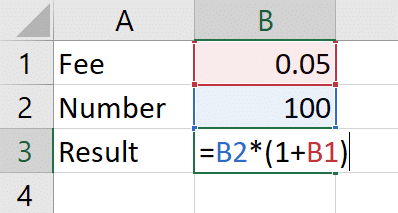# Why is change calculated with *(1+B1) and not just *B1?

In several of our lessons, we deal with absolute references and/or calculating changes to a value.

With these topics, the example typically is about adding or subtracting a percentage amount from a number.

We often forget it but this is simply calculated with basic math.

If you want to subtract 10% from the number 100, how do you do that?

You could multiply the number with 0.9, which is the equivalent of calculating 90% of the number.

The formula in Excel would look like this: =100*0.9

But this requires you to calculate the 0.9 with mental arithmetic.

What about subtracting 32% from 100?

That’s… 100 … eeeh… multiplied by 0.78…. No, 0.68!

It takes a brief moment to calculate it yourself. And that’s a waste of time when you’re sitting in front of the world’s most potent calculator (I’m talking about Excel).

Is there another way to calculate this more easily?

You can simply let Excel do the subtraction.

You’re looking to create this formula: =100*0.68

The 0.68 (68%) is the share of the number that’s left after you’ve subtracted the 0.32 (32%) from 1 (100%).

To calculate this directly in Excel, just put it in a parenthesis: =100*(1-0.32)

That’s easy, right?

Usually, the percentage you want to subtract or add from the number is put in a cell.

In the picture below, we want to add the fee, 0.05 (5%) to the number 100.If the formula didn’t have the 1+, the result would be 5.

But the example tries to calculate the number after the fee is added.

So, the result should be (and is) 105.